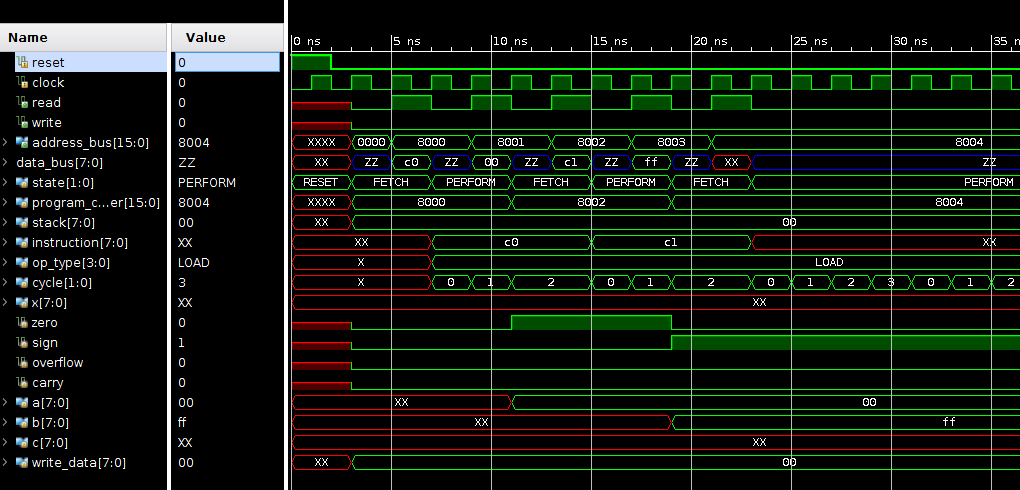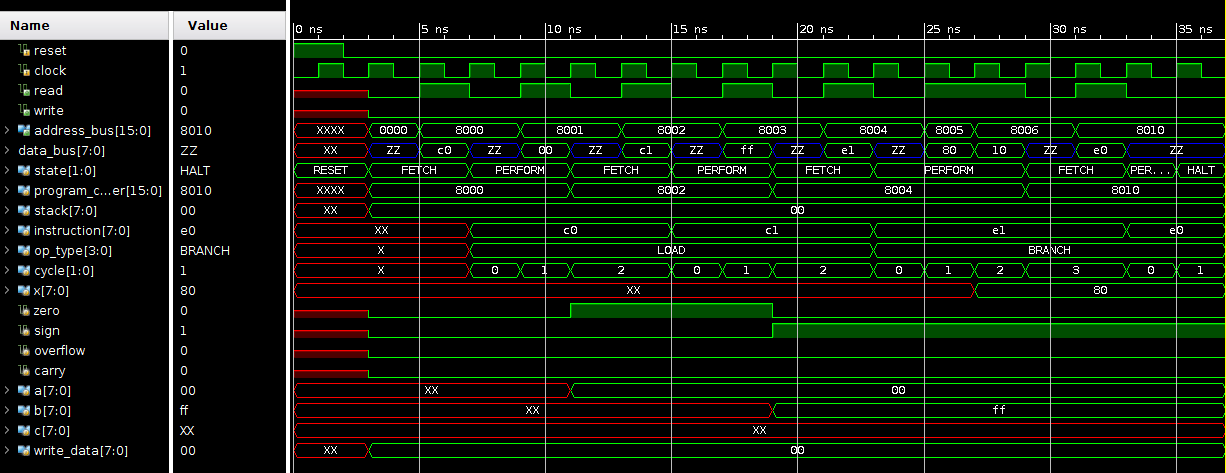# Beginning Logic Design – Part 12

Hello and welcome to Part 12 of my Beginning Logic Design series! In the last post I implemented the `LOAD` and `STORE` sets of operations. In this round I will start to implement branching operations that allow the code to take different paths through a program.

# Branching Out

One of the most important things a CPU needs is the ability to branch, or jump, to different program code based on some conditions. Imagine an if/else statement in most common programming languages. You have some condition that you evaluate, and based on that outcome you perform one set of operations or another.

The first step I want to take towards implementing this is adding my CPU flags that will represent the conditions that can be considered.

1. // CPU flags
2. logic zero;
3. logic sign;
4. logic overflow;
5. logic carry;

I’ll also modify my CPU’s `RESET` state to set these all to `0` on reset.

1. RESET: begin
2. state <= FETCH;
3. program_counter <= 'h8000;
4. stack <= 0;
5. read <= 0;
6. write <= 0;
7. address_bus <= 0;
8. write_data <= 0;
9. zero <= 0;
10. sign <= 0;
11. overflow <= 0;
12. carry <= 0;
13. end

Next, I want to check to make sure the instructions I have defined so far are setting these flags as I’d like them to. Right now the only commands that should be modifying these flags are the `LOAD` commands, if the number loaded is `0`, then the `zero` flag should be set. If the number loaded could be interpreted  as a negative number (it’s highest bit is `1`), the `sign` flag should get set.

This is easily implement by adding this to the final cycle of each load command, right near where the register loaded is being set.

1. if (data_bus == 0)
2. zero <= 1;
3. else
4. zero <= 0;
5. sign <= data_bus;

I’ll write a test program to load `A` with `0`, then load `B` it with `ff` (-1).

1. c0 00
2. c1 ff

In simulation, it looks good!Now for the `BRANCH` operations themselves! For now I have 10 Branch operations I’d like to define:

1. 0 - Halt
2. 1 - Jump
3. 2 - Branch if zero set
4. 3 - Branch if zero unset
5. 4 - Branch if sign set
6. 5 - Branch if sign unset
7. 6 - Branch if overflow set
8. 7 - Branch if overflow unset
9. 8 - Branch if carry set
10. 9 - Branch if carry unset

These will be fairly quick to implement, as all of them are quite similar. First I will setup my overall `case` statement structure.

1. BRANCH: begin
2. case (instruction[3:0])
3. // Halt
4. 0: begin
5. end
6. // Jump
7. 1: begin
8. end
9. // Branch zero set
10. 2: begin
11. end
12. // Branch zero clear
13. 3: begin
14. end
15. // Branch sign set
16. 4: begin
17. end
18. // Branch sign clear
19. 5: begin
20. end
21. // Branch overflow set
22. 6: begin
23. end
24. // Branch overflow clear
25. 7: begin
26. end
27. // Branch carry set
28. 8: begin
29. end
30. // Branch carry clear
31. 9: begin
32. end
33. endcase
34. end

The `HALT` operation is dead simple, just change the CPU state to `HALT`

1. // Halt
2. 0: begin
3. state <= HALT;
4. end

I’ll give this operation a test shortly, first I want to implement my `JUMP` operation. The implementation of that begins pretty similarly to the other operations that look for a memory address, there will be 3 cycles. The first address byte will be requested; on the second cycle the first byte read and the second byte requested; on the last cycle the reading will stop and the `program_counter` will be set to its new value.

1. // Jump
2. 1: begin
3. case (cycle)
4. 0: begin
5. read <= 1;
6. address_bus <= program_counter + 1;
7. end
8. 1: begin
9. address_bus <= program_counter + 2;
10. x <= data_bus;
11. end
12. 2: begin
13. read <= 0;
14. program_counter <= {x,data_bus};
15. state <= FETCH;
16. end
17. endcase
18. end

Now, as a test, I’ll extend my last program that set the flags to include a `JUMP` call, after that instruction I’ll pad a few bytes with `FF` and at `8010` I’ll have my `HALT` instruction.

1. c0 00
2. c1 ff
3. e1 80 10
4. ff ff ff ff ff ff ff ff ff
5. e0

Testing it in the simulator it works like a charm!## Conditional Branches

The conditional branches are fairly simple to implement, I just took my `JUMP` implementation and added a condition on the flag during the first cycle. If the condition is not met, we can modify the program counter to start the fetch of the next instruction

1. // Branch zero set
2. 2: begin
3. case (cycle)
4. 0: begin
5. if (zero) begin
6. read <= 1;
7. address_bus <= program_counter + 1;
8. end else begin
9. program_counter += 3;
10. state <= FETCH;
11. end
12. end
13. 1: begin
14. address_bus <= program_counter + 2;
15. x <= data_bus;
16. end
17. 2: begin
18. read <= 0;
19. program_counter <= {x,data_bus};
20. state <= FETCH;
21. end
22. endcase
23. end

This is basically the same for the `Branch zero clear` operation, the only change is a flipping of the `if`/`else` statements.

1. // Branch zero clear
2. 3: begin
3. case (cycle)
4. 0: begin
5. if (zero) begin
6. program_counter += 3;
7. state <= FETCH;
8. end else begin
9. read <= 1;
10. address_bus <= program_counter + 1;
11. end
12. end
13. 1: begin
14. address_bus <= program_counter + 2;
15. x <= data_bus;
16. end
17. 2: begin
18. read <= 0;
19. program_counter <= {x,data_bus};
20. state <= FETCH;
21. end
22. endcase
23. end

The remaining operations are a very slight derivation of these two, some copy-pasta will do the trick and the only change is what flag is being looked at in the `if` condition.

1. // Branch sign set
2. 4: begin
3. case (cycle)
4. 0: begin
5. if (sign) begin
6. read <= 1;
7. address_bus <= program_counter + 1;
8. end else begin
9. program_counter += 3;
10. state <= FETCH;
11. end
12. end
13. 1: begin
14. address_bus <= program_counter + 2;
15. x <= data_bus;
16. end
17. 2: begin
18. read <= 0;
19. program_counter <= {x,data_bus};
20. state <= FETCH;
21. end
22. endcase
23. end
24. // Branch sign clear
25. 5: begin
26. case (cycle)
27. 0: begin
28. if (sign) begin
29. program_counter += 3;
30. state <= FETCH;
31. end else begin
32. read <= 1;
33. address_bus <= program_counter + 1;
34. end
35. end
36. 1: begin
37. address_bus <= program_counter + 2;
38. x <= data_bus;
39. end
40. 2: begin
41. read <= 0;
42. program_counter <= {x,data_bus};
43. state <= FETCH;
44. end
45. endcase
46. end
47. // Branch overflow set
48. 6: begin
49. case (cycle)
50. 0: begin
51. if (overflow) begin
52. read <= 1;
53. address_bus <= program_counter + 1;
54. end else begin
55. program_counter += 3;
56. state <= FETCH;
57. end
58. end
59. 1: begin
60. address_bus <= program_counter + 2;
61. x <= data_bus;
62. end
63. 2: begin
64. read <= 0;
65. program_counter <= {x,data_bus};
66. state <= FETCH;
67. end
68. endcase
69. end
70. // Branch overflow clear
71. 7: begin
72. case (cycle)
73. 0: begin
74. if (overflow) begin
75. program_counter += 3;
76. state <= FETCH;
77. end else begin
78. read <= 1;
79. address_bus <= program_counter + 1;
80. end
81. end
82. 1: begin
83. address_bus <= program_counter + 2;
84. x <= data_bus;
85. end
86. 2: begin
87. read <= 0;
88. program_counter <= {x,data_bus};
89. state <= FETCH;
90. end
91. endcase
92. end
93. // Branch carry set
94. 8: begin
95. case (cycle)
96. 0: begin
97. if (carry) begin
98. read <= 1;
99. address_bus <= program_counter + 1;
100. end else begin
101. program_counter += 3;
102. state <= FETCH;
103. end
104. end
105. 1: begin
106. address_bus <= program_counter + 2;
107. x <= data_bus;
108. end
109. 2: begin
110. read <= 0;
111. program_counter <= {x,data_bus};
112. state <= FETCH;
113. end
114. endcase
115. end
116. // Branch carry clear
117. 9: begin
118. case (cycle)
119. 0: begin
120. if (carry) begin
121. program_counter += 3;
122. state <= FETCH;
123. end else begin
124. read <= 1;
125. address_bus <= program_counter + 1;
126. end
127. end
128. 1: begin
129. address_bus <= program_counter + 2;
130. x <= data_bus;
131. end
132. 2: begin
133. read <= 0;
134. program_counter <= {x,data_bus};
135. state <= FETCH;
136. end
137. endcase
138. end

That’ll do it for the basic branching operations! In the next post I will begin the implementation of  the operations that will utilize the ALU. As always, I welcome your feedback and questions in the comments. Keep tinkering!

Posted on Categories Digital Logic Design